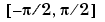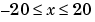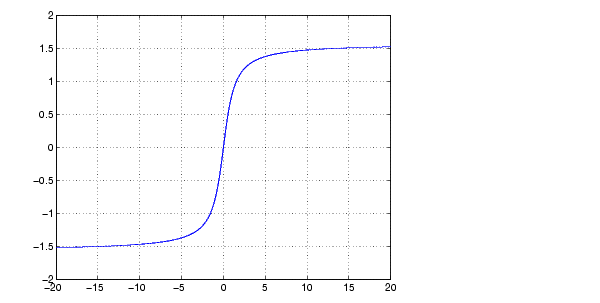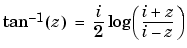MATLAB Function Referenceatan

Inverse tangent, result in radians

Syntax

• ```Y = atan(X)
```

Description

```Y = atan(X) ``` returns the inverse tangent (arctangent) for each element of `X`. For real elements of `X`, `atan(X)` is in the range.

The `atan` function operates element-wise on arrays. The function's domains and ranges include complex values. All angles are in radians.

Examples

Graph the inverse tangent function over the domain.

• ```x = -20:0.01:20;
plot(x,atan(x)), grid on```

Definition

The inverse tangent can be defined as

•Algorithm

`atan` uses FDLIBM, which was developed at SunSoft, a Sun Microsystems, Inc. business, by Kwok C. Ng, and others. For information about FDLIBM, see http://www.netlib.org.

See Also

`atan2`, `tan`, `atand`, `atanh`

© 1994-2005 The MathWorks, Inc.